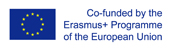This project has been funded with support from the European Commission.
This web site reflects the views only of the author, and the Commission cannot be held responsible for any use which may be made of the information contained therein.

Select language   >   IT EN RO PL LT

### Probability

Subject taught
- Math

Type of Product
- Videos

Language Skills Developed
- Listening
- Speaking

Transferable/Scientific Skills Developed
Student will develop self-motivation, analytical skills, problem-solving skills

Description
General aims: To give an introduction to teachers and students how to construct an area model to find every possible outcome of an experiment.
The video presents hands-on probability model activity that involves building an area model which can be used as a visual representation multiple probability scenarios involving candy.
Student will be able to determine the probability of an event using the results of an experiment, give an example of mutually exclusive events. Student will develop positive learning habits such as organisational and problem-solving skills. The teacher will have a useful example how to show students processes and develop, use, and evaluate probability models.

Linguistic aims:
- to read and listen English with ease
- to understand content
- to communicate experiences
- to learn from written texts
- to master tools for gathering information
- to express understanding and experiences
- to enable students to understand topic correctly
- to translate words connected with probability in English into the mother tongue using one’s own words.

Subject specific aims:
- to familiarise students with the ways in which we talk about uncertainty
- to engage students in activities which will give them contact with the ideas of probability
- student is able to determine the probability of an event using the results of an experiment
- student is able to give the example of mutually exclusive events
-student is able to construct an area model to find every possible outcome of an experiment .

Target group age:
Students from primary school (12-14 years old), before using the resource they need to know how to do basic operation with fractions and percentages. What is more, this video can be used by grade teachers who wants to teach probability using English.

Level of competence in English (CEFR)
B1/B1+

Time required to use the resource with the students:
The teacher can use the resource with the students for one lessons. Video lasts 7 minutes.

How to use it
Before using the video during one’s classes, the students should be able to do basic operation with fractions and percentages.
The teacher asks students to watch the video and does pauses in certain moments to give students opportunity to make notes, asks questions and develop some problems presented in the video.
During lessons the teacher should ask the student in English:

“What is an experiment?” “Give the examples of experiments.”
“What kind of experiment was presented in the video?”
“What does it mean that events are mutually exclusive?”
“What is a probability model?”
“How can I help myself understand probability?”
The students give the answers with the examples. The teacher should give them a feedback and show students more examples on the black table. The teacher highlights some new words for students in English and students should find the translation these words into the mother tongue and write down the new words in English in the notebook with the right translation in their own words.

Students should do similar exercises which involve constructing an area model and practice them in pairs. The teacher gives a feedback to the students and helps them determine the probability of given examples if they need it.
As a homework students can create their own exercises and determine the probability of an event using the results of the experiment.

Possible difficulties for the students
Student who has poor knowledge of English can have problems with understanding the content. Student who has problems doing basic operations with fractions can face difficulties in solving math problems with probability.